Test Description

## 10 Questions MCQ Test | Olympiad Test: Integers -2

Olympiad Test: Integers -2 for Class 7 2023 is part of Class 7 preparation. The Olympiad Test: Integers -2 questions and answers have been prepared according to the Class 7 exam syllabus.The Olympiad Test: Integers -2 MCQs are made for Class 7 2023 Exam. Find important definitions, questions, notes, meanings, examples, exercises, MCQs and online tests for Olympiad Test: Integers -2 below.
Solutions of Olympiad Test: Integers -2 questions in English are available as part of our course for Class 7 & Olympiad Test: Integers -2 solutions in Hindi for Class 7 course. Download more important topics, notes, lectures and mock test series for Class 7 Exam by signing up for free. Attempt Olympiad Test: Integers -2 | 10 questions in 20 minutes | Mock test for Class 7 preparation | Free important questions MCQ to study for Class 7 Exam | Download free PDF with solutions
 1 Crore+ students have signed up on EduRev. Have you?
Olympiad Test: Integers -2 - Question 1

### 72÷? = –4

Detailed Solution for Olympiad Test: Integers -2 - Question 1

72 ÷ x = – 4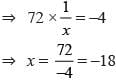Olympiad Test: Integers -2 - Question 2

### What is the product of additive inverse of 7 and multiplicative inverse of 14?

Detailed Solution for Olympiad Test: Integers -2 - Question 2

Additive inverse of 7 = –7
Multiplicative inverse of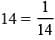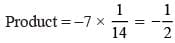Olympiad Test: Integers -2 - Question 3

### [16–{5+(2–7)}]–[12–{7–(3–4)}]?

Detailed Solution for Olympiad Test: Integers -2 - Question 3

[16 – {5 + (2 – 7)}] – [12 – {7 – (3 – 4)}]
= [16 – {5 – 5}] – [12 – {7 + 1}]
= [16 – 0] – [12 – 8] = 16 – 4 = 12

Olympiad Test: Integers -2 - Question 4

[16÷{11–(2+5)}] =?

Detailed Solution for Olympiad Test: Integers -2 - Question 4

[16 ÷ {11 – (2 + 5)}]
= [16 ÷ {11 – 7}] = [ 16 ÷ 4] = 4

Olympiad Test: Integers -2 - Question 5

25+16÷4×3–5+7 of (–3) = ?

Detailed Solution for Olympiad Test: Integers -2 - Question 5

25+16 ÷ 4×3 –5+7 of (–3)
= 25 + 16 ÷ 4×3 –5 + (–21)
= 25 + 4×3 –5 –21
= 25 + 12–26
= 37 – 26 = 11

Olympiad Test: Integers -2 - Question 6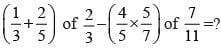Detailed Solution for Olympiad Test: Integers -2 - Question 6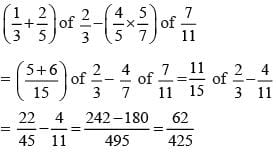Olympiad Test: Integers -2 - Question 7

A cement company earns a profit of  Rs 8 per bag of white cement sold and a loss of Rs 5  per bag of grey cement sold. The company sells 3000 bags of white cement and 500 bags of grey cement in a month. What is its profit or loss?

Detailed Solution for Olympiad Test: Integers -2 - Question 7

Profit by selling white cement = 8×3000 = 24000
Loss by selling grey cement = 5×5000 = 25000
Loss = 25000 – 24000 = 1000

Olympiad Test: Integers -2 - Question 8

In a class test containing 15 questions 4 marks are given for every correct answer and (–2) marks are given for every incorrect answer. Mohan attempts all questions but only 9 of his answers are correct. What is his total score?

Detailed Solution for Olympiad Test: Integers -2 - Question 8

Marks for 9 correct answers = 9 × 4 = 36
Marks for 6 incorrect answers = 6 × (–2) = –12
Mohan’s total score = 36 + (–12) = 36 –12 = 24

Olympiad Test: Integers -2 - Question 9

A shopkeeper earns a profit of Rs 1 by selling one copy and incure a loss of 40 paise per pen while selling pens of his old stock. In a particular month he incurs a loss of Rs 5. In this period he sold 45 copy. How many pens did he sell in this period?

Detailed Solution for Olympiad Test: Integers -2 - Question 9

Profit earned by selling 45 copies = Rs 45
Total loss = Rs 5 Profit earned + loss incurred = Total loss.
⇒ 45 + Loss incurred = –5
⇒ Loss incurred = –5 – 45 = –50 = – 5000 Paise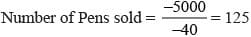Olympiad Test: Integers -2 - Question 10

The temperature at 12 noon was 10°C per hour until midnight. At what time would the temp be 8°C below zero?

Detailed Solution for Olympiad Test: Integers -2 - Question 10

10°C + x = – 8°
After 9 hours means at 9 P.M.
Temp will be –8°C.

 Use Code STAYHOME200 and get INR 200 additional OFF Use Coupon Code